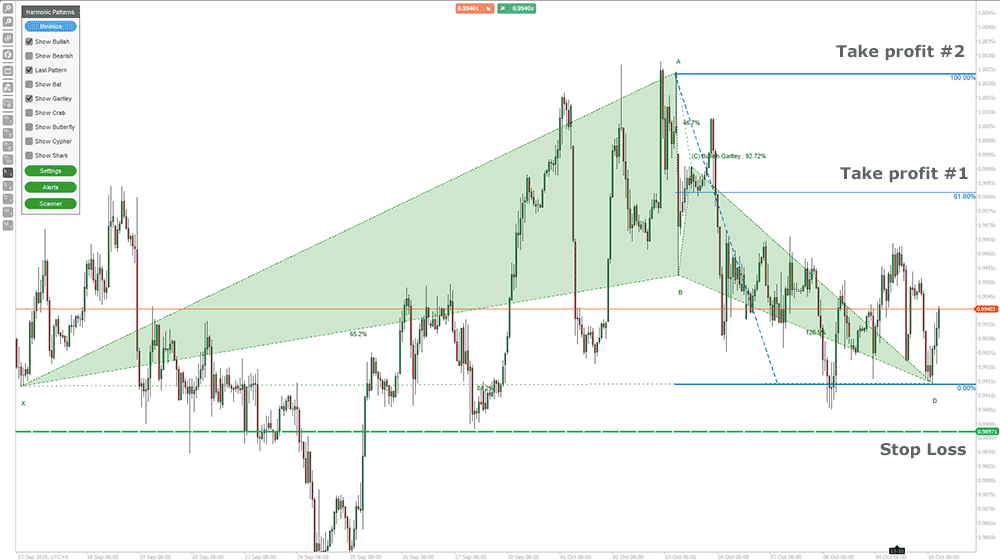You can now identify Gartley patterns using the cTrader trading platform, the Gartley Harmonic Pattern is a retracement pattern that usually forms near the swing highs and lows in ideal conditions which involves 5 pivot or swings points.

The Gartley pattern is the most popular harmonic chart pattern and they work on the assumption that Fibonacci sequences can be used to build geometric structures, such as breakouts and retracements in the prices. The two most common uses of Fibonacci numbers in the financial markets used with retracements and extensions, Fibonacci retracements measure how far a pullback could go. Fibonacci extensions measure how far an impulse wave could go.

## The Golden Ratio in Forex

The Golden ratio is a special number found by dividing a line into two parts so that the longer part divided by the smaller part is also equal to the whole length divided by the longer part. It is often symbolized using phi, after the 21st letter of the Greek alphabet.

a/b = (a+b)/a = 1.6180339887498948420 …

1.618 inverse equals 0.618 or 61.8%